# RD Sharma Solutions For Class 7 Maths Chapter 16 Congruence Exercise 16.5

RD Sharma Solutions for Class 7 Maths Exercise 16.5 Chapter 16 Congruence are available here. The expert faculty at BYJU’S have formulated solutions based on the marks allotted for each step of a problem. The main objective is to help students in solving problems, in turn, which makes the subject easier to understand. Our experts have provided chapter-wise solutions which help students perform well in the exam. Students can download the RD Sharma Solutions for Class 7 for further reference. This exercise explains RHS congruence condition for two congruent triangles.

## Download the PDF of RD Sharma Solutions For Class 7 Maths Chapter 16 – Congruence Exercise 16.5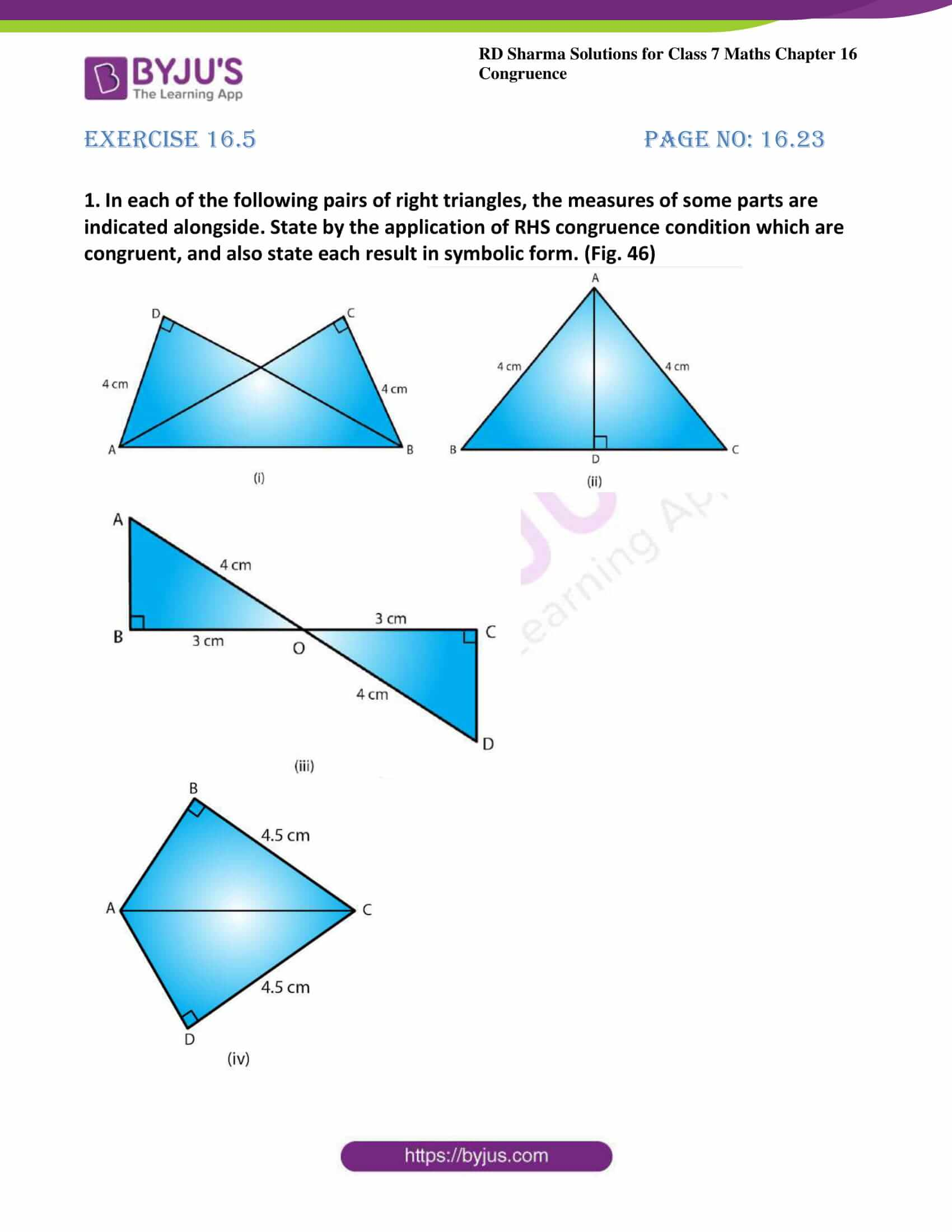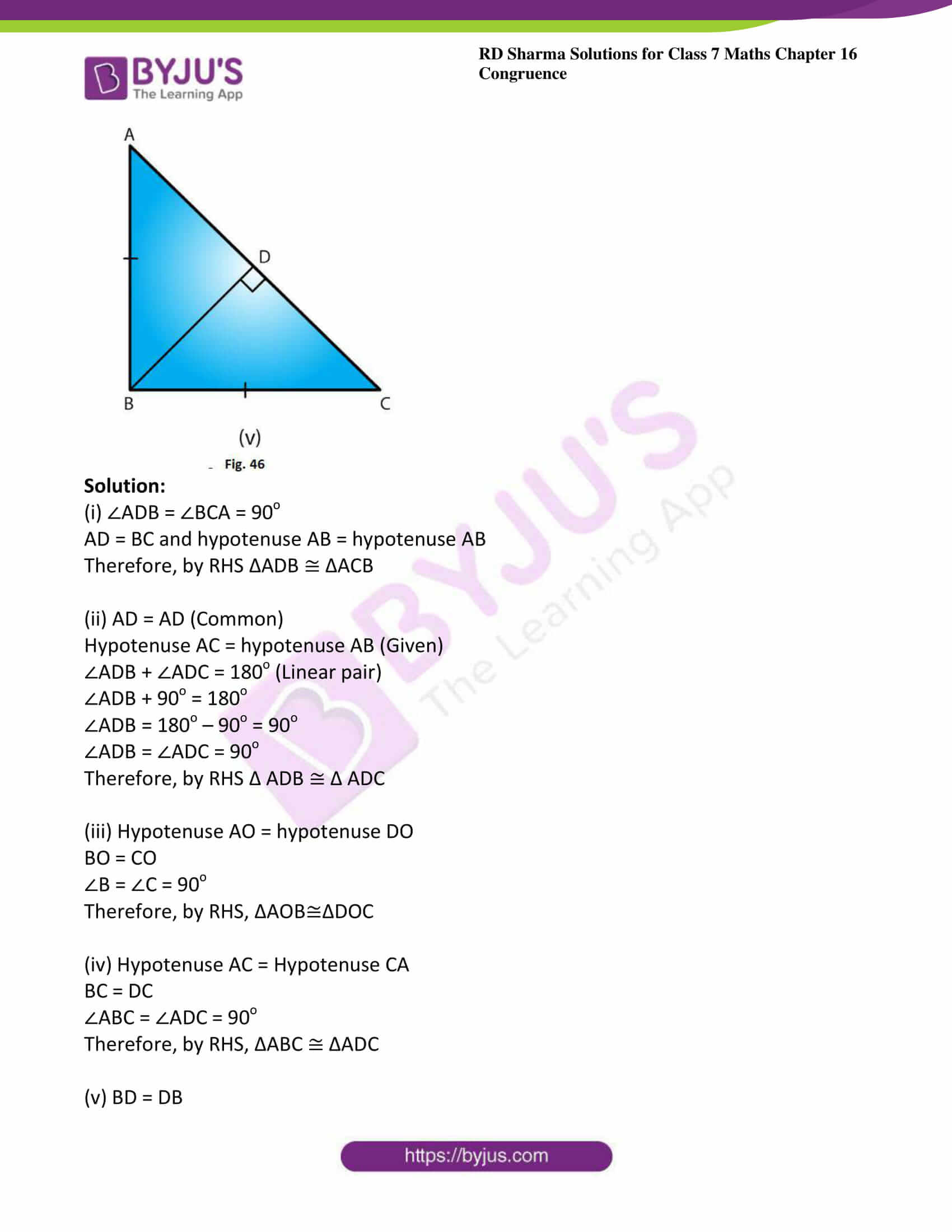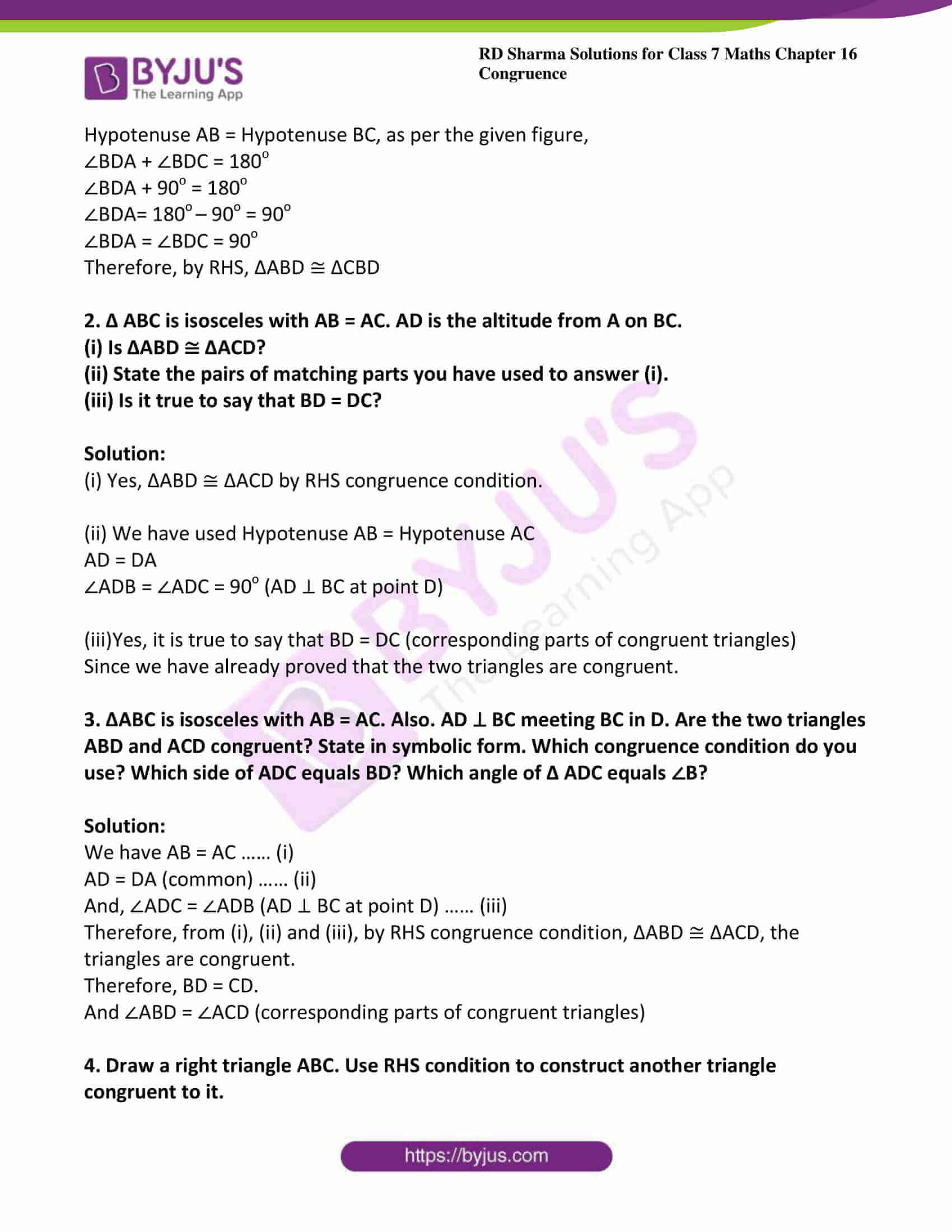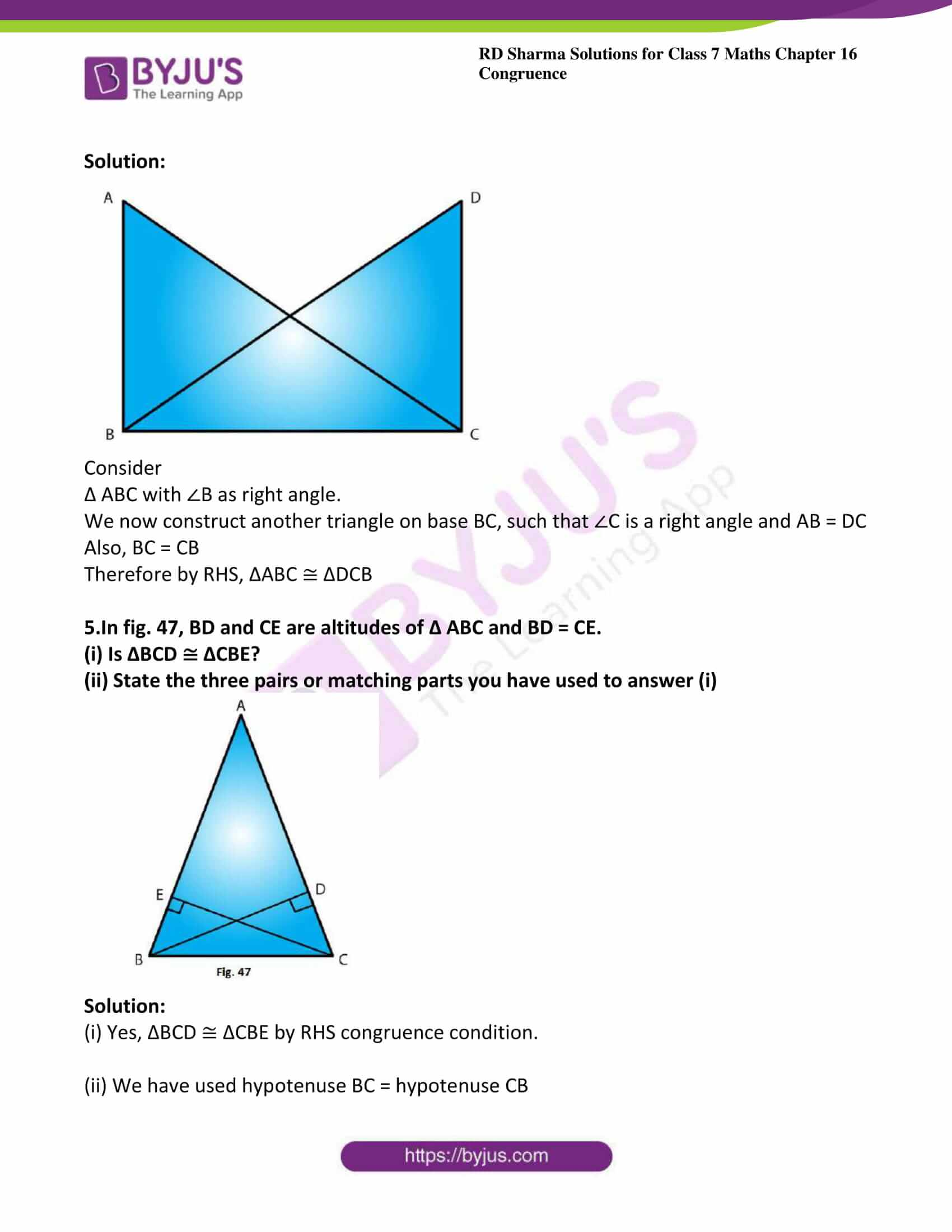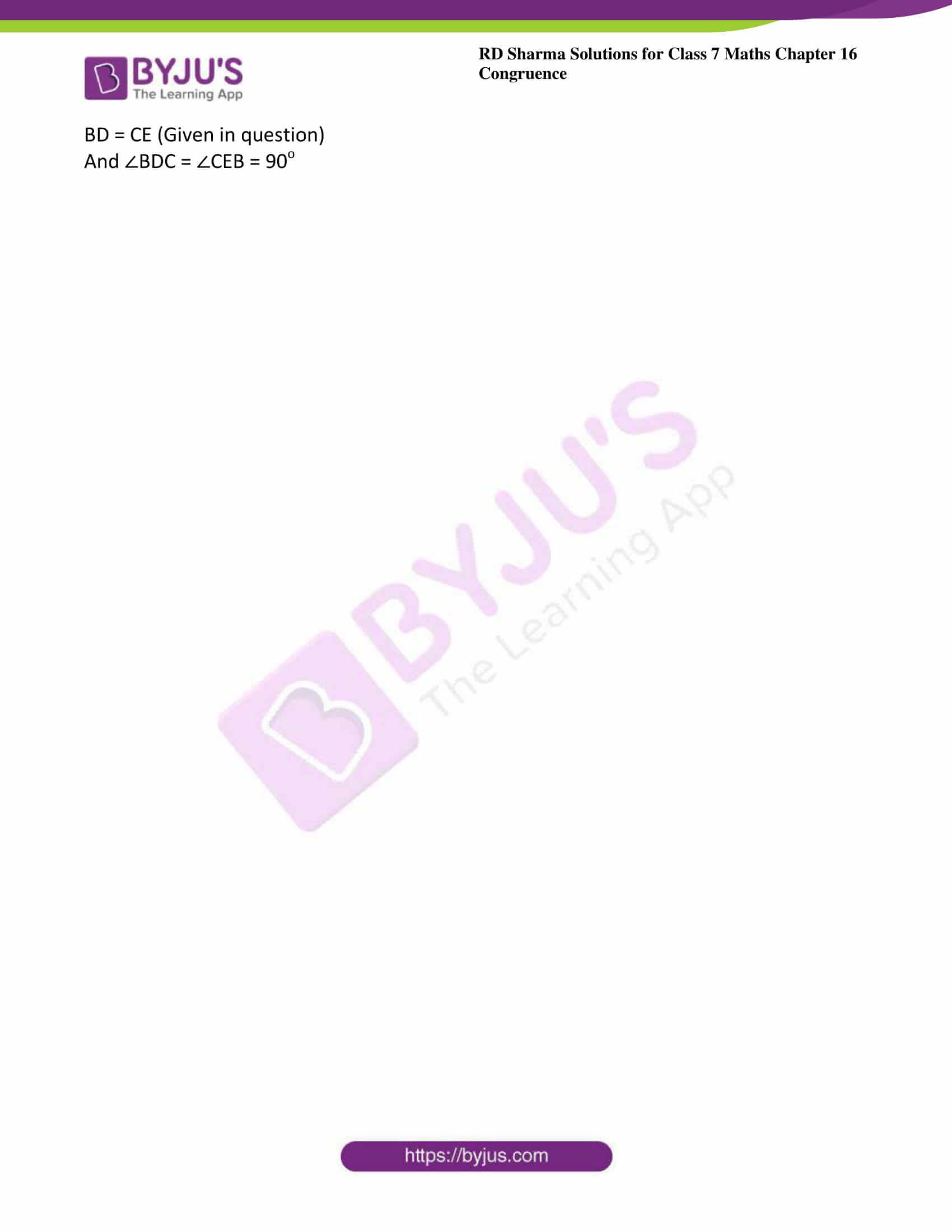### Access answers to Maths RD Sharma Solutions For Class 7 Chapter 16 – Congruence Exercise 16.5

Exercise 16.5 Page No: 16.23

1. In each of the following pairs of right triangles, the measures of some parts are indicated alongside. State by the application of RHS congruence condition which are congruent, and also state each result in symbolic form. (Fig. 46)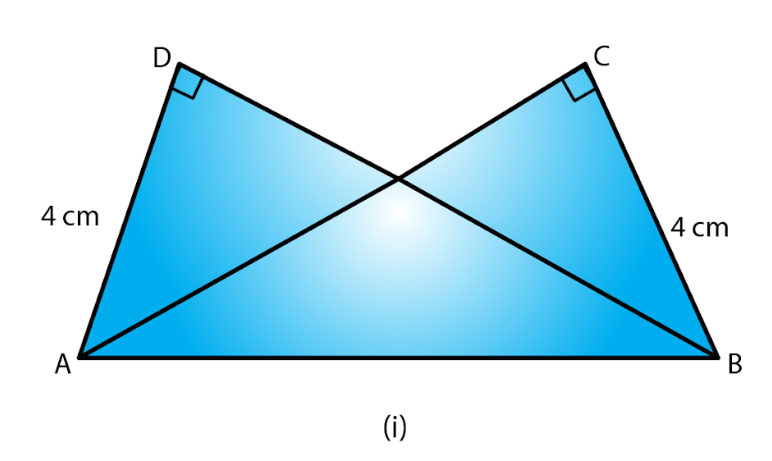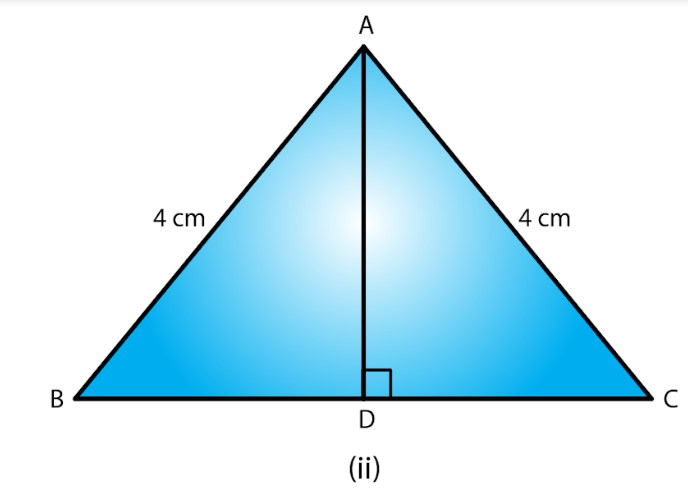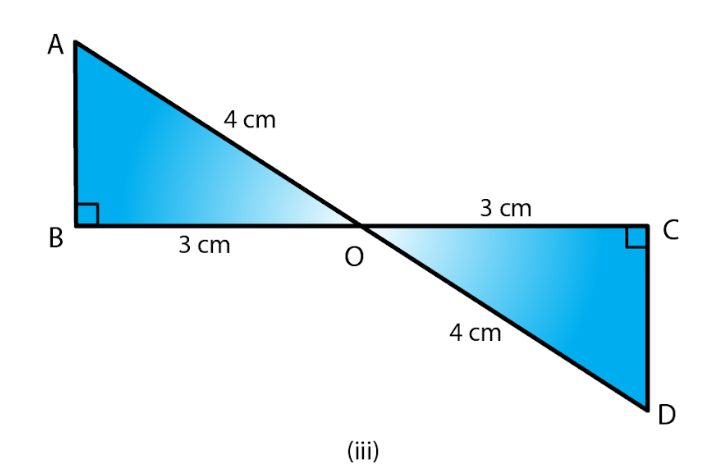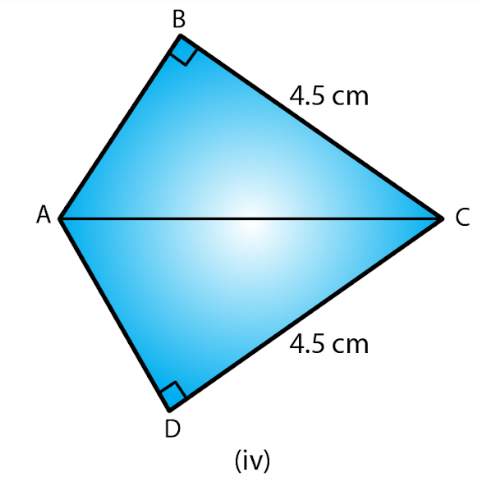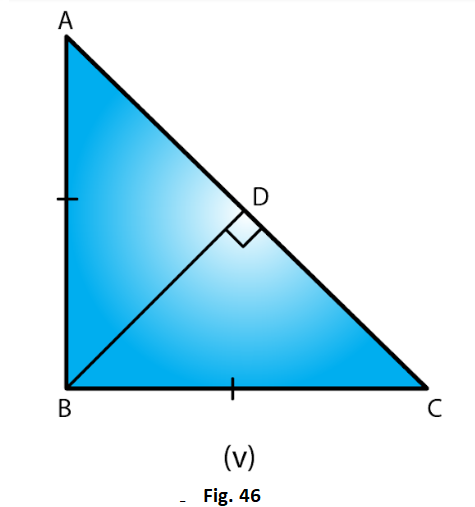Solution:

(i) ∠ADB = ∠BCA = 90o

AD = BC and hypotenuse AB = hypotenuse AB

Therefore, by RHS ΔADB ≅ ΔACB

Hypotenuse AC = hypotenuse AB (Given)

∠ADB = 180o – 90o = 90o

(iii) Hypotenuse AO = hypotenuse DO

BO = CO

∠B = ∠C = 90o

Therefore, by RHS, ΔAOB≅ΔDOC

(iv) Hypotenuse AC = Hypotenuse CA

BC = DC

Therefore, by RHS, ΔABC ≅ ΔADC

(v) BD = DB

Hypotenuse AB = Hypotenuse BC, as per the given figure,

∠BDA + ∠BDC = 180o

∠BDA + 90o = 180o

∠BDA= 180o – 90o = 90o

∠BDA = ∠BDC = 90o

Therefore, by RHS, ΔABD ≅ ΔCBD

2. Δ ABC is isosceles with AB = AC. AD is the altitude from A on BC.

(i) Is ΔABD ≅ ΔACD?

(ii) State the pairs of matching parts you have used to answer (i).

(iii) Is it true to say that BD = DC?

Solution:

(i) Yes, ΔABD ≅ ΔACD by RHS congruence condition.

(ii) We have used Hypotenuse AB = Hypotenuse AC

(iii)Yes, it is true to say that BD = DC (corresponding parts of congruent triangles)

Since we have already proved that the two triangles are congruent.

3. ΔABC is isosceles with AB = AC. Also. AD ⊥ BC meeting BC in D. Are the two triangles ABD and ACD congruent? State in symbolic form. Which congruence condition do you use? Which side of ADC equals BD? Which angle of Δ ADC equals ∠B?

Solution:

We have AB = AC …… (i)

AD = DA (common) …… (ii)

Therefore, from (i), (ii) and (iii), by RHS congruence condition, ΔABD ≅ ΔACD, the triangles are congruent.

Therefore, BD = CD.

And ∠ABD = ∠ACD (corresponding parts of congruent triangles)

4. Draw a right triangle ABC. Use RHS condition to construct another triangle congruent to it.

Solution: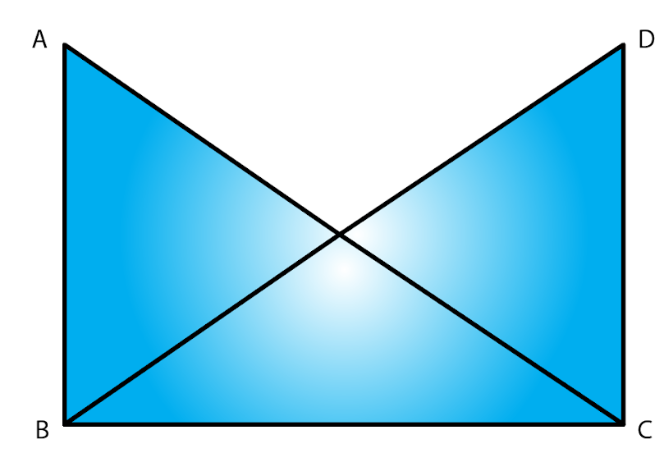Consider

Δ ABC with ∠B as right angle.

We now construct another triangle on base BC, such that ∠C is a right angle and AB = DC

Also, BC = CB

Therefore by RHS, ΔABC ≅ ΔDCB

5.In fig. 47, BD and CE are altitudes of Δ ABC and BD = CE.

(i) Is ΔBCD ≅ ΔCBE?

(ii) State the three pairs or matching parts you have used to answer (i)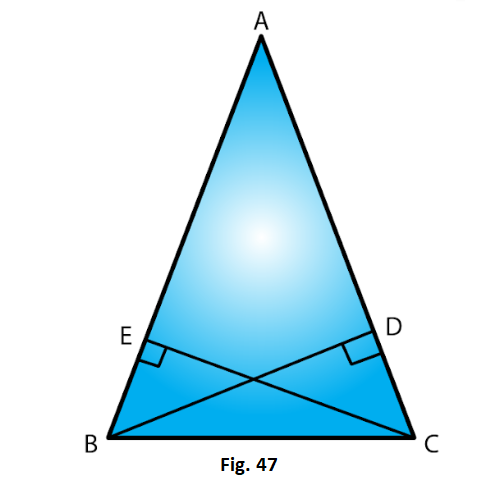Solution:

(i) Yes, ΔBCD ≅ ΔCBE by RHS congruence condition.

(ii) We have used hypotenuse BC = hypotenuse CB

BD = CE (Given in question)

And ∠BDC = ∠CEB = 90o